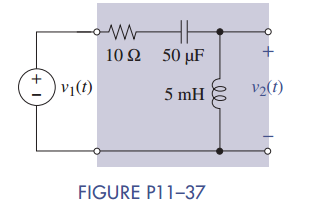### Create an Account

Home / Questions / The circuit in Figure P11 37 is in the steady state with v1t 25 cos 2000t V Find v2SS(t)...

# The circuit in Figure P11 37 is in the steady state with v1t 25 cos 2000t V Find v2SS(t). Where are the poles located Without doing any calculations repeat for

The circuit in Figure P11–37 is in the steady state with v1(t) = 25 cos 2000t V. Find v2SS(t). Where are the poles located? Without doing any calculations repeat for v(t)= 5 V.May 24 2020 View more View LessSubscribe To Get Solution